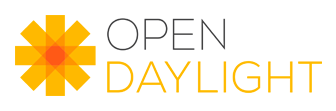index 76bfb75..12fbb1b 100644 (file)
@@ -9,9 +9,37 @@ package org.opendaylight.controller.model.api.type;
\r
import org.opendaylight.controller.yang.model.api.ConstraintMetaDefinition;\r
\r
+/**\r
+ * The Range Constraint interface is used to restrict integer and decimal\r
+ * built-in types, or types derived from those.\r
+ * <br>\r
+ * A range consists of an explicit value consists of an explicit value, or a lower bound\r
+ * returned by {@link #getMin()} and an upper bound returned by\r
+ * {@link #getMax()}. <br>\r
+ * <br>\r
+ * Each explicit value and range boundary value given in\r
+ * the range expression MUST match the type being restricted, or be one of the\r
+ * special values "min" or "max". "min" and "max" mean the minimum and maximum\r
+ * value accepted for the type being restricted, respectively\r
+ * <br>\r
+ * <br>\r
+ * This interface was modeled according to definition in <a\r
+ * href="https://tools.ietf.org/html/rfc6020#section-9.2.4">[RFC-6020] The\r
+ * range Statement</a>.\r
+ */\r
public interface RangeConstraint extends ConstraintMetaDefinition {\r
\r
-    Long getMin();\r
+    /**\r
+     * Returns the length-restricting lower bound value.\r
+     * \r
+     * @return the length-restricting lower bound value.\r
+     */\r
+    Number getMin();\r
\r
-    Long getMax();\r
+    /**\r
+     * Returns the length-restricting upper bound value.\r
+     * \r
+     * @return the length-restricting upper bound value.\r
+     */\r
+    Number getMax();\r
}\r©2013 OpenDaylight, A Linux Foundation Collaborative Project. All Rights Reserved.
OpenDaylight is a registered trademark of The OpenDaylight Project, Inc.
Linux Foundation and OpenDaylight are registered trademarks of the Linux Foundation.
Linux is a registered trademark of Linus Torvalds.